# How to Count Cells That Contain Specific Text in Excel

This post will guide you how to count cells that contain specific text in Excel. How do I count cells with text and characters using a formula in Excel. How to count cells with specific text value using VBA Macro in Excel.

## Count Cells with Specific Text using Formula

Assuming that you have a big range of data (B1:B5), and you need to count cells that contain specific text (excel). You can use a formula based on the COUNTIF function to count cells with specific text value in Excel. Like this:

`=COUNTIF(\$B\$1:\$B\$5,"*excel*")`

Type this formula into a blank cell and press Enter key on your keyboard.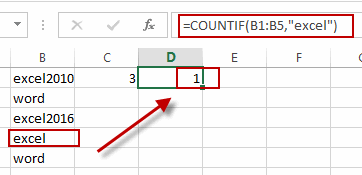This formula will count the number of cells in the range B1:B5 that contain “excel” text string by matching the content of each cell against the pattern “*excel*”. And the asterisk symbol is a wildcard that means “matching any number of characters”.

If you only want to count cells that are an exact match, and you can use the following formula to count the cells with exact “excel” text string. Like this:

`=COUNTIF(B1:B5,”excel”)`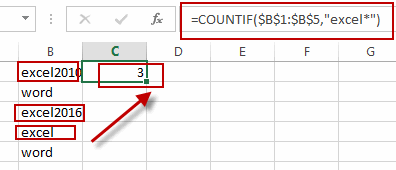## Count Cells with Specific Text using VBA

You can also use an Excel VBA Macro to achieve the same result of counting the number of cells with specific text value. Here are the steps:

#1 open your excel workbook and then click on “Visual Basic” command under DEVELOPER Tab, or just press “ALT+F11” shortcut.#2 then the “Visual Basic Editor” window will appear.

#3 click “Insert” ->”Module” to create a new module.#4 paste the below VBA code  into the code window. Then clicking “Save” button.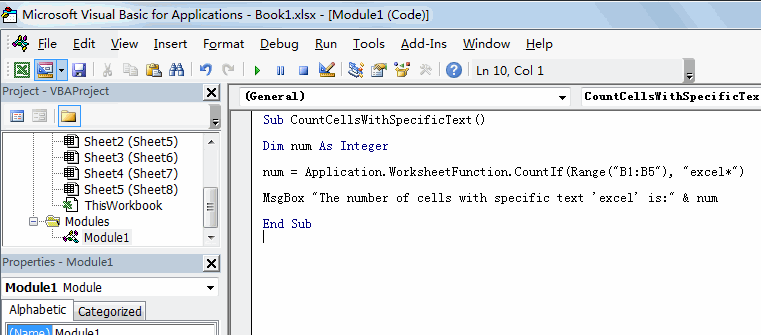```Sub CountCellsWithSpecificText()
Dim num As Integer
num = Application.WorksheetFunction.CountIf(Range("B1:B5"), "excel*")
MsgBox "The number of cells with specific text 'excel' is:" & num
End Sub```

Note: you should change the range as you need, and also need to change the mattern as you need.

#5 back to the current worksheet, then run the above excel macro. Click Run button.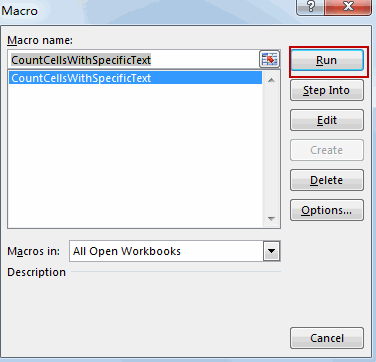#6 Lets see the result: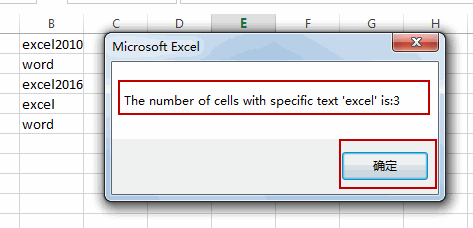### Related Functions

• Excel COUNTIF function
The Excel COUNTIF function will count the number of cells in a range that meet a given criteria. This function can be used to count the different kinds of cells with number, date, text values, blank, non-blanks, or containing specific characters.etc.= COUNTIF (range, criteria)…

Sidebar